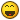# VIXRA paper on Newtonian G

## Recommended Posts

It is argued in this [very] short paper (almost too short to take seriously in a way) an argument that the Newtonian G can be replaced for the Planck length.

Dimensionally, the equation stands up, what about the physical meaning? This is no less an attempt to quantize G in such a way that it conforms with modern Planck scale physics. Of course, assume we are working in the Planck space, then the curvature also has to satisfy Planckian dynamics. Of course, I have found my own ways to deal with this - the quantum vacuum is about non-commutativity and this can be fully expressible through the spacetime uncertainty relation, in which it is a reinterpretation of the usual energy-time uncertainty. That relationship holds as

$R_{\mu \nu} \geq \frac{1}{\ell^2}$

The paper is too short in my opinion to be classed as a true ''study'' into how Planck dynamics should enter general relativity. It feels more theoretical than anything based on experimental physics.

##### Share on other sites

lol Anytime someone refers strictly to his own papers as support I immediately question. To state the paper is too short is an understatement. The problem is in Planck units G=1 it is already in natural units before even using the equation above. Or rather it appears he is mixing natural and cgs units for G.

c=g=h=1

his notes on the first references support that. In order to derive the natural units in the first place.

Edited by Mordred
##### Share on other sites

I'll be honest, I haven't looked at what units he was working in. Probably best not to see it just as ''G'' the whole object is the upper limit of the classical gravitational force and he seems to be wanting to reinterpret it in terms of some Planck dynamics. So he writes

$\frac{8 \pi \ell}{mc^2}$

$F_{upper}\ell = mc^2$

and

$F_{upper} = \frac{mc^2}{\ell}$

inverse is

$\frac{1}{F_{upper}} = \frac{mc^2}{\ell}$

So I don't know, just looks like a dimensional argument, nothing special.

##### Share on other sites

Well he has done something odd ball see equation 3 of this article showing the EFE as per natural units and his equation 2

the latter paper gives it as

$R_{\mu\nu}-\frac{1}{2}Rg_{\mu\nu}+\Lambda g_{\mu\nu}=\frac{8\pi}{m^2_p}T_{\mu\nu}$

where he gives $\frac{8\pi l_p}{m_pc^2}T_{\mu\nu}$

Edited by Mordred
##### Share on other sites

We circle around the constants. I practiced a lot## Create an account

Register a new account